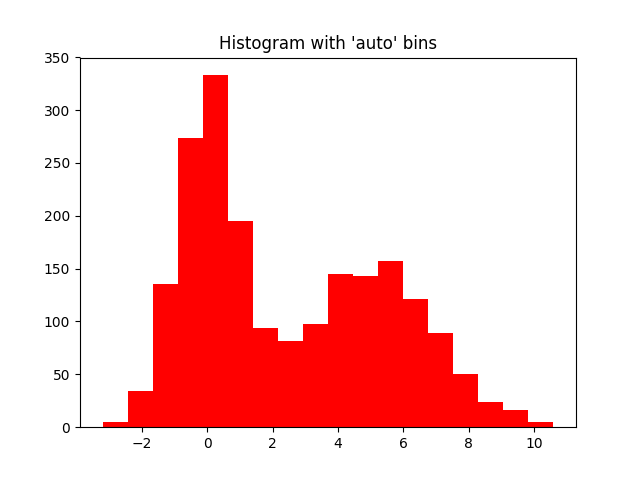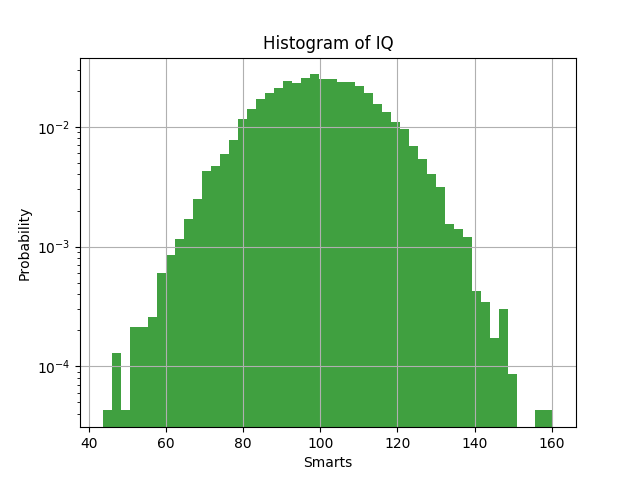# Histogram in Matplotlib – Data Visualization using Python

A histogram is an accurate representation of the distribution of numerical data. It is an estimate of the probability distribution of a continuous variable (quantitative variable)

A histogram is a plot that lets you discover, and show, the underlying frequency distribution (shape) of a set of continuous data. This allows the inspection of the data for its underlying distribution (e.g., normal distribution), outliers, skewness, etc.

To construct a histogram from a continuous variable you first need to split the data into intervals, called bins. In the example, age has been split into bins, with each bin representing a 10-year period or 5-year period.

### Histograms are based on area, not height of bars

In a histogram, it is the area of the bar that indicates the frequency of occurrences for each bin. This means that the height of the bar does not necessarily indicate how many occurrences of scores there were within each individual bin. It is the product of height multiplied by the width of the bin that indicates the frequency of occurrences within that bin

### What is the difference between a bar chart and a histogram?

The major difference is that a histogram is only used to plot the frequency of score occurrences in a continuous data set that has been divided into classes, called bins. Bar charts, on the other hand, can be used for a great deal of other types of variables including ordinal and nominal data sets.

Let us see how to plot histogram using python and maplotlib

Output is as belowHere is another example with additional details